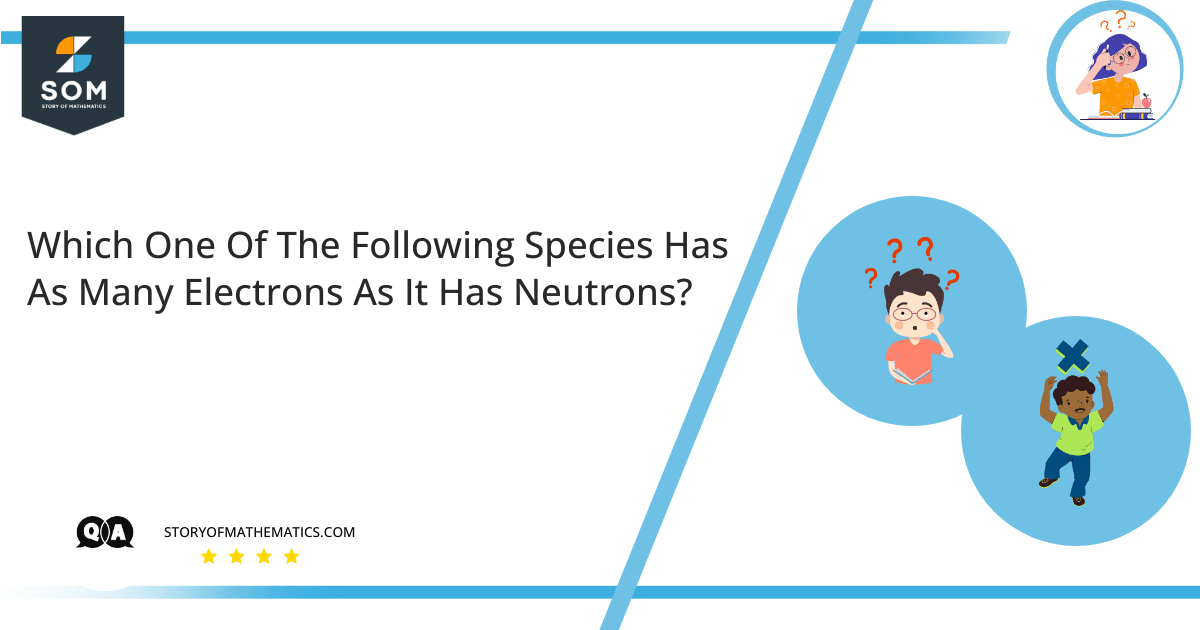# Which one of the following species has as many electrons as it has neutrons?– Select the correct answer from the following options:

– $(a) \ ^{14}C$

– $(b) \ ^{14}C^{2+}$

– $(c) \ ^{40}{\rm Ca}^{2+}$

– $(d) \ ^1H$

– $(e) \ ^{19}F^-$

An atom consists of three Subatomic Particles. The Center of an Atom or Nuclei has Protons and Neutrons whereas the Electrons are revolving around the nuclei. Protons are positively charged particles and Electrons are negatively charged particles. Neutrons are electrically neutral. For an electrically neutral atom, the number of protons is equal to the number of electrons.

$Number\ of\ Protons=Number\ of\ Electrons$

Atomic Number $Z$ of an element is the number of protons present in the nuclei of its atom.

Mass Number $A$ is the sum of the number of protons and neutrons.

$Mass\ Number=Number\ of\ Neutrons+Number\ of\ Protons$

Or:

$Mass\ Number=Number\ of\ Neutrons+Atomic\ Number\ Z$

$A=N+Z$

An element is scientifically represented by the following symbol:

${_Z^A}{\ X}^{\ q}$

Where:

$A=$ Mass Number

$Z=$ Atomic Number

$q=$ Electric Charge on an atom

Option (a) – $\ ^{14}C$

Atomic Number of Carbon $C=6$

As per standard notation:

${_6^{14}}C$

The Number of Protons and Number of Electrons is $6$ each.

Mass Number $A$ is $14$, so:

$A=N+Z$

$14=N+6$

$N=8$

Hence, the Number of Electrons is not equal to the Number of Neutrons.

Option (b) – $\ ^{14}C^{2+}$

Atomic Number of Carbon $C=6$

As per standard notation:

${_6^{14}}C^{2+}$

The Number of Protons is $6$. Since the atom has a $2+$ positive charge, the Number of Electrons is:

$E=6-2=4$

Mass Number $A$ is $14$, so:

$A=N+Z$

$14=N+6$

$N=8$

Hence, the Number of Electrons is not equal to the Number of Neutrons.

Option (c) – $\ ^{40}{\rm Ca}^{2+}$

Atomic Number of Calcium $Ca=20$

As per standard notation:

${_{20}^{40}}{\rm Ca}^{2+}$

The Number of Protons is $20$. Since the atom has a $2+$ positive charge, hence the Number of Electrons is:

$E=20-2=18$

Mass Number $A$ is $40$, so:

$A=N+Z$

$40=N+20$

$N=20$

Hence, the Number of Electrons is not equal to the Number of Neutrons.

Option (d) – $\ ^1H$

Atomic Number of Hydrogen $H=1$

As par standard notation:

${_1^1}H$

The Number of Protons and Number of Electrons is $1$ each.

Mass Number $A$ is $1$, so:

$A=N+Z$

$1=N+1$

$N=0$

Hence, the Number of Electrons is not equal to the Number of Neutrons.

Option (e) – $\ ^{19}F^-$

Atomic Number of Fluorine $F=9$

As per standard notation:

${_9^{19}}F^-$

The Number of Protons is $9$. Since the atom has a $-1$ negative charge, the Number of Electrons is:

$E=9+1=10$

Mass Number $A$ is $19$, so:

$A=N+Z$

$19=N+9$

$N=10$

Hence, the Number of Electrons is equal to the Number of Neutrons.

## Numerical Result:

Option (e) is correct. $\ ^{19}F^-$

## Example

Calculate the Number of Electrons and Neutrons in $\ ^{24}{\rm Mg}^{2+}$.

Atomic Number of Magnesium $Mg=12$

As par standard notation:

${_{12}^{24}}{\rm Mg}^{2+}$

The Number of Protons is $12$. Since the atom has a $2+$ positive charge, the Number of Electrons is:

$E=12-2=10$

Mass Number $A$ is $24$, so:

$A=N+Z$

$24=N+12$

$N=24$